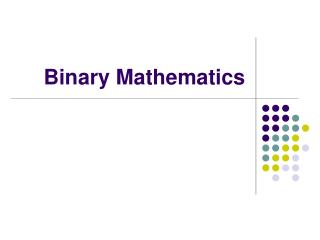DownloadDownload PresentationBinary Mathematics

# Binary Mathematics

Download Presentation## Binary Mathematics

- - - - - - - - - - - - - - - - - - - - - - - - - - - E N D - - - - - - - - - - - - - - - - - - - - - - - - - - -
##### Presentation Transcript

1. Binary Mathematics

2. Counting system • There are three kinds of people in the world: those who can count, and those who can not. - Unknown Wisdom • Today’s class • Numbering system • Conversion between 10 based and 2 based numbering system. • Binary Mathematics. • Quiz

3. Base 10 counting system • We happened to use the current counting system, because we happened to have ten fingers. • If dinosaurs had ruled the earth, they would be happy to use a 8-based counting system.

4. Numbers • Ancient Africa • Notches on a bone. • Egyptians/Roman • Each magnitude is represented by a symbol. • Indian/Arabian (Modern numbering system) 1,475,268

5. Base 10 (Decimal numbers) • What does 157 mean? • 157 = 1 x 100 + 5 x 10 + 7 x 1 = 1 x 102 + 5 x 101 + 7 x 100

6. Binary Code • Imagine a specie that only has two fingers. how can they count? • A computer is such kind of two-finger specie. 0 and 1 • Each place is the exponential of 2

7. Base 10 vs Base 2 Base 10 157 157 = 1 x 100 + 5 x 10 + 7 x 1 = 1 x 102 + 5 x 101 + 7 x 100 Base 2 1011 = 1 x 23 + 0 x 22 + 1 x 21 + 1 x 20 1011 = 1 x 8 + 0 x 4 + 1 x 2 + 1 x 1

8. Binary Bits and Bytes • 1 bit is a single bit of information, a 1 or 0 • Only two possible values • 1 byte is 8 bits, an 8 bit word • 256 possible values from 0-255 base 10 or 00000000 to 11111111 base 2 • 10100110 is a single byte

9. Base 10 to Binary

10. Binary mathematics • 0+0=0 • 1+0=1 • 1+1=10

11. Hexadecimal (base 16) • Binary code is too long in representation. Hex is much shorter. • Converting a binary number to a Hex number is relatively easy • Every 4 bit can convert to a Hex • Problem: we are short of numbers • A-10 B-11 C-12 D-13 E-14 F-15

12. Lookup table

13. Example

14. Wisdom said • There are 10 kinds of people in the world, those who use binary counting system, and those who don’t.

15. Quiz • No Calculators!!!! • Convert binary code to Decimal number. • 10100101 (Bin) • Convert Decimal number to binary code • 176 (Dec) • Convert Hexadecimal number to binary • BADDEF • Add these two binary numbers • 10001101+11011100=?

16. Answer • 10100101 (Binary) = 165 (Decimal) • 176 (Decimal)= 10110000 (Binary) • BADDEF=1011,1010,1101,1101,1110,1111 • The result of summation • 101101001